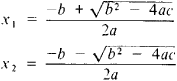Also found in: Dictionary, Thesaurus.

(mathematics)
Any second-degree polynomial equation.
McGraw-Hill Dictionary of Scientific & Technical Terms, 6E, Copyright © 2003 by The McGraw-Hill Companies, Inc.
The following article is from The Great Soviet Encyclopedia (1979). It might be outdated or ideologically biased.

an equation of the form ax2 + bx + c = 0, where a, b, and c are any number and are called the coefficients of the equation. A quadratic equation has two roots, which are found by the formulasThe expression D = b2 − 4ac is called the discriminant of the quadratic equation. If D > 0, then the roots of the quadratic equation are real and unequal; if D < 0, then the roots areconjugate complex numbers; if D = 0, then the roots are real andequal. The Vièta formulas x1 + x2 = − b/a and x\x2 = c/a link the roots and coefficients of a quadratic equation. The left-hand side of a quadratic equation can be expressed in the form α (xx2) (xx2). The function y = ax2 + bx + c is called a quadratic trinomial, and its graph is a parabola with the vertex at the point M (−b/2a; cb2/4a) and axis of symmetry parallel to the. y-axis; the direction of the branches of the parabola coincides with the sign of a. The solution of the quadratic equation was already known in geometric form to ancient mathematicians.

References in periodicals archive ?
where the first equality is obtained from the relationships in (3.7) and Vieta's formula for the quadratic equation (3.6), and the third equality from the fact that [n.sub.2] is one root of the quadratic equation (3.6) and Vieta's formula for the quadratic equation (3.6).
Elementary math facts and the sounds of letters are obvious choices, but any information that is needed with high frequency is a candidate - in algebra, that's the quadratic equation.
Finally, the quadratic equation (1.1) is generalized Ulam-Hyers-Rassias stable.
The quadratic equation (3.1) is ([psi],[gamma])-stable if and only if [PQ.sub.[psi],[gamma]](G, E) = Q(G, E).
So [m.sub.1] and [m.sub.2] satisfy the quadratic equation
Table 1 Quadratic equation Factors Solutions [x.sup.2] - 4x + 0 = 0 (x - 4)(x - 0) Two real solutions: x = 4 and x = 0 [x.sup.2] - 4x + 3 = 0 (x - 3)(x - 1) Two real solutions: x = 3 and x = 1 [x.sup.2] - 4x + 4 = 0 (x - 2)(x - 2) = One real solution: [(x - 2).sup.2] x = 2 [x.sup.2] - 4x + 5 = 0 No real factors Two complex solutions: x = 2 + i and x = 2 - i [x.sup.2] - 4x + 8 = 0 No real factors Two complex solutions: x = 2 + 2i and x = 2 - 2i [x.sup.2] - 4x + 13 = 0 No real factors Two complex solutions: x = 2 + 3i and x = 2 - 3i When the quadratic equation [x.sup.2] - 4x + 5 is solved using the quadratic formula, the discriminant is -4.
The first intersection of the quadratic equation with the LRP plateau was obtained by the equation L = [[beta].sub.0] + [[beta].sub.1]P[B.sub.i] + [[beta].sub.2]P[B.sub.i.sup.2], and the CP level corresponding to the intersection was calculated by the expression (-[[beta].sub.1] + ([[beta].sub.1.sup.2] - 4 [[beta].sub.2] [([[beta].sub.0] - L)).sup.1/2])/(2[[beta].sub.2]).
The first author treating the stability of the quadratic equation was F.
There are many geometrical approaches to the solution of the quadratic equation with real coefficients.
Consider the quadratic equation with the generally complex coefficients shown in Equation (6).
In order to give some meaning to the concepts discussed above, attention will now be focused on the quadratic equation originally presented in Equation (3), which yields generally complex roots.

Site: Follow: Share:
Open / Close